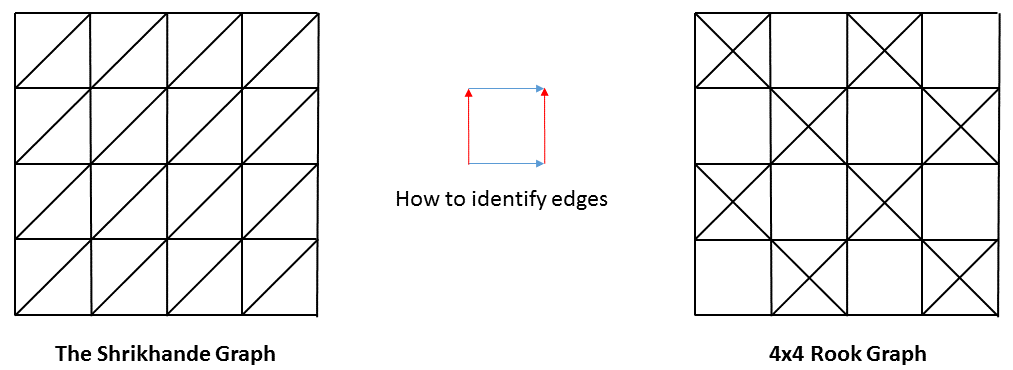## August 2016 solution

Although this month (as an exception) no proofs were required, two solvers, Jim Boyce and Oscar Volpatti, did send proofs of claims related to the riddle, demonstrating the rich structure underpinning this simple-looking problem.

Both sent me essentially-identical proofs relating to Part 2 which I will quote here (in Boyce's formulation), as their proof surely rates in the top ten list of the most elegant proofs I've ever seen.

Oscar Volpatti sent an additional proof regarding Part 1 and follow-up computed example graphs.

Thank you, both Jim and Oscar, for making the solution so much more interesting!

#### Part 1:

It is possible to construct a party acquaintance graph with k=1 for any odd n value. This is done by joining (n-1)/2 triangles at a single vertex.

Oscar Volpatti gives the following proof that no solution exists for any even n.

Take an arbitrary node, a, and consider all its neighbours. Because each neighbour has exactly one other neighbour of a as a neighbour of its own (it is their single joint neighbour), the subgraph induced by the neighbours of a is a perfect matching. Therefore, their number is even. We conclude that the degree of every node is even. Let N be the set of a's neighbours.

Next, take another arbitrary node, xa. It must have exactly one common neighbour with a, so there is exactly one edge leading from N to x. Summing these over all x we have n-1 and adding the |N| edges from N to a we see that there is a total of |N|+n-1 half-edges from N. (Where by "half-edges" we mean that we count an edge from N to outside of N as 1 but a node from N to N as 2. Note that this must be an even number because it is the sum of the degrees of all nodes in N, each of which is even. Given that |N| is also even (being the degree of a), we conclude that n is odd.

Q.E.D.

#### Part 2:

There are, in fact, a grand total of three party acquaintance graphs with k=2 (excluding those with n<2). These are the 4-clique, the 4-by-4 rook graph and the Shrikhande graph.

The following is Jim Boyce's proof that no other such graphs exist. It assumes that we've already discovered all graphs for small n by exhaustive searching, which is easy to do due to the many constraints on the design of the graph.

Once again let a be an arbitrary vertex and N be its neighbours. Let d=|N|. There is a bijection between the set of all vertices other than a and the set of pairs of neighbours of a: if we take two nodes u and v in N by definition they must have exactly two common neighbours, so a and some other node x that is by this uniquely defined; on the other hand, every node xa has exactly two neighbours in common with a, so the pair {u,v} is by this also uniquely defined. The fact that the relation {u,v} ↔ x is a bijection indicates that the two sets have the same cardinality. The total number of vertices in the graph is therefore 1+d(d-1)/2, and, furthermore, the graph is regular.

Let A be the adjacency matrix of the graph. The "all 1's'' vector is clearly an eigenvector with eigenvalue d. This is the only eigenvector with an eigenvalue as large (in magnitude) as d.

Consider A2. A2[i,j] counts the number of paths of length 2 from i to j (via some other vertex, k). So A2[i,j] is d if i=j, and is 2 otherwise. So A2 is (d-2)*I + 2*(the all 1's matrix).

Let v be some other eigenvector, with eigenvalue m. v is also an eigenvector of A2, with eigenvalue m2. Since v is orthogonal to "all 1's", (A2)v = (d-2)*I*v + 0. So, m2 = d-2.

The Trace of A is 0. This is the sum of the eigenvalues of A. The eigenvalues of A are m (repeated i times), -m (repeated j times), and d (once).

If m (= √d-2) is irrational, (i-j)*m + d is not 0, so Tr(A) is not 0 -- a contradiction.

If m is rational, it is an integer. Tr(A) = 0 = i*m-j*m+m2+2. So, m is a factor of 2; it is either 1 or 2, and d is either 3 or 6.

Q.E.D.

Oscar Volpatti continued from here programmatically to generate one example party acquaintance graph with k=4 (the graph whose nodes are the binary strings of length 6 and the edges are between nodes of Hamming distance 1) and one with k=6 (the graph whose nodes are the binary strings of length 5 that have even Hamming weight and the edges are the nodes of Hamming distance 2).

My final thank-you of the month goes to Lorenz Reichel, who managed to find a graphical embedding of both the 4-by-4 Rook graph and the Shrikhande graph so as to emphasise their basic similarities and differences.

Both drawings are toroidal embeddings: in order to see them properly, you will need to print them on a piece of paper and then fold the paper back so that the right and left side of the drawing are glued together from behind. This will make your paper into a cylinder. Next, take the top and the bottom and fold them back, gluing them together, so the whole shape becomes a doughnut.(*)

(*) "Using your Head is Permitted" is generally in favour of going paper-less, but does not recommend readers to try and fold their monitor screens into doughnut shapes.

Here are Lorenz's drawings.Many thanks to Jim, Oscar and Lorenz, as well as to Dominic van der Zypen who sent the riddle in.

Readers wishing for an extra challenge should try to organise a party whose acquaintance graph is the Shrikhande graph...